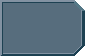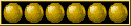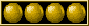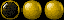A question

A question

What is this 12.75 x 106 TeraWatts
what does this equal in watts, terawatts is this 12.75 exawatts
CrewmanPosts: 4
Joined: Fri Oct 08, 2010 3:11 am

Re: A question

You mean 12.75 x 10 to the power of 6 TW, I take it.

That would be 12,750,000 TW, or 12,750 PetaWatts, 12.75 ExaWatts.
Give a man a fire, and you keep him warm for a day. SET a man on fire, and you will keep him warm for the rest of his life...Graham KennedyPosts: 11274
Joined: Mon Jul 09, 2007 1:28 pm
Location: Banbury, UK

Re: A question

The usual way of representing it on a forum would be 12.75 x 10^6, which makes the OP a typo (the ^ is above the 6).
email, ergo spamIanKennedyPosts: 5321
Joined: Mon Jul 09, 2007 1:28 pm
Location: Oxford, UK

Re: A question

I see it put that way in a lot of star trek manuals and it is hard to understand what the number is
CrewmanPosts: 4
Joined: Fri Oct 08, 2010 3:11 am

Re: A question

Like 2.7x10^8 terawatt

Is that 2.7 exawatts?
CrewmanPosts: 4
Joined: Fri Oct 08, 2010 3:11 am

Re: A question

admiral horton wrote:Like 2.7x10^8 terawatt

Is that 2.7 exawatts?

No, it's 270,000,000 TW, which is 270 EW.
Only two things are infinite - the universe and human stupidity, and I'm not sure about the universe: Albert Einstein.Captain SeafortPosts: 15487
Joined: Thu Jul 19, 2007 12:44 pm
Location: Blighty

Re: A question

admiral horton wrote:Like 2.7x10^8 terawatt

Is that 2.7 exawatts?

It goes :

10^6 = Mega
10^9 = Giga
10^12 = Tera
10^15 = Peta
10^18 = Exa
10^21 = Zetta
10^24 = Yotta

In each case the number after the ^ is the number of zeroes after a 1. So 3 x 10^6 is 3 x 1,000,000 <- six zeroes. And 5x10^9 is 5 x 1,000,000,000 < nine zeroes.
Give a man a fire, and you keep him warm for a day. SET a man on fire, and you will keep him warm for the rest of his life...Graham KennedyPosts: 11274
Joined: Mon Jul 09, 2007 1:28 pm
Location: Banbury, UK

Re: A question

Ok thanks I think it is getting clearer.
So anything between 10^7 & 10^8 would still be in the mega range
CrewmanPosts: 4
Joined: Fri Oct 08, 2010 3:11 am

Re: A question

10^7 would be 10M and 10^8 would be 100M.

The best way of thinking of it is to move the decimal place ^n times to the right. So:

1.234 x 10^4 => 12340.
12.323 x 10^5 => 1232300.

The other way you see it represented in on a calculator, where it will be shown as an EE button. On a calculator you would enter the number by typing

1.234EE4

rather than typing

1.234 x 10 xy 4

In fact if you enter it that way it will not produce the correct answers as the operator precedence rules will cause your equations to go very very wrong.

The number format is generally called scientific notation. You normally stick to only one digit before the decimal place so 12.323 x 10^5 would normally be written as 1.2323 x 10^6. The exception to that is when units are involved. There are letters and names that represent powers in multiples of 3. As Graham said:

10^3 = Kilo (k - lower case to distinguish from Kelvin)
10^6 = Mega (M)
10^9 = Giga (G)
10^12 = Tera (T)
10^15 = Peta (P)
10^18 = Exa (E)
10^21 = Zetta (Z)
10^24 = Yotta (Y)

Sticking to these multiples causes you to break the rules for only one digit before the decimal place:

24 kilometres => 24 x 10^3 metres => 24000 metres.

8231232 metres => 8.231232 x 10^6 metres => 8.231232 Megametres
email, ergo spamIanKennedyPosts: 5321
Joined: Mon Jul 09, 2007 1:28 pm
Location: Oxford, UK

Re: A question

admiral horton wrote:Ok thanks I think it is getting clearer.
So anything between 10^7 & 10^8 would still be in the mega range

Only if the number of units is singular.

So 5*10^7 Watts = 50 MegaWatts

But 5*10^7 MegaWatts = 50 TeraWatts

The reason a person might write it as 5*10^7 MegaWatts is because they are trying to compare it to something that uses between 1 and 999 MegaWatts, or because they didn't look up proper scientific notation before writing it down.
Relativity Calculator
My Nomination for "MVAM Critic Award" (But can it be broken into 3 separate pieces?)
Coalition
Lieutenant CommanderPosts: 967
Joined: Tue Dec 02, 2008 3:34 am
Location: Georgia, United States

Re: A question

It's highly unusual, if not wrong, to write 5*10^7 MegaWatts in science. Mega indicates 10^6 and you already have 10^7 there, which equates to 5*10^13 Watts, or using the rule of ^3, 50 * 10^12 = 50 TeraWatts. The only conceivable reason I can think of is if you had a relatively lay audience and wanted them to be able to do a direct comparison using a fixed unit, for example 50 MegaWatts and 50 million MegaWatts.

You should either stick to 5 x 10^7 Watts vs 5 x 10^13 Watts or use 50 MegaWatts vs 50 TerraWatts.
email, ergo spamIanKennedy